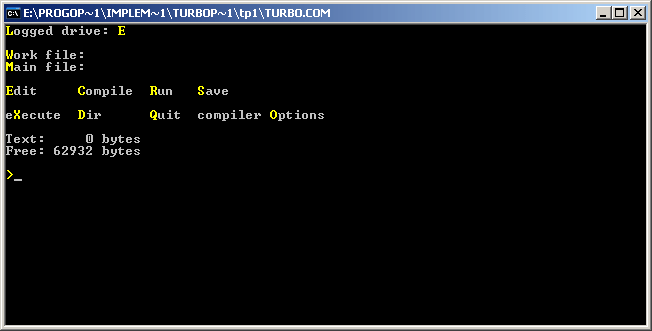# Turbo Pascal 1.0

Version of implementation Turbo Pascal of programming language Pascal

Turbo Pascal 1.0 was the first version of Turbo Pascal series, released on November 20, 1983.

Turbo Pascal 1.0 is available for PC-DOS and CP/M operating systems, both very popular at the time of its creation. It requires only 64kb of RAM, compiles code to .COM or .CHN executables of at most 64kb, supports CGA graphics mode and allows sound manipulation using PC Speaker.

This version doesn’t possess the distinctive blue full-screen user interface with pull-down menus, for which the later Turbo-products are recognized. Instead it features a text-based menu screen, as shown on the screenshot. Available menu commands include:

• Edit: enter full-screen editor with work file loaded in it.
• Save: saves currently loaded work file.
• Compile: compile work file. There exist three compile modes — “memory” (store compiled program in memory), “COM-file” and “CHN-file” (create executable file of corresponding type).
• Run: run the compiled program, if there is one in memory. If the program was last compiled to a file, it can’t be run from Turbo Pascal.
• Execute: “Sorry, eXecute is not yet implemented in 8086/88 versions”.
• Dir: lists the contents of current directory.
• Compiler options: allows to choose between compile modes and tune each of them.
• Quit: quits Turbo Pascal.## Examples:

### Hello, World! - Pascal (57):

``````program helloworld;

begin
writeln('Hello, World!');
end.
``````

### Factorial - Pascal (96):

This example is exactly the same as main recursive example for Pascal implementations, except for that it uses `real` data type to store factorial values. Command `writeln(f:-1:0)` outputs the floating point number `f` with 0 digits after decimal point and left-justifies it.

``````program factorial;

function fact(n: integer): real;
begin
if (n = 0) then
fact := 1
else
fact := n * fact(n - 1);
end;

var
n: integer;

begin
for n := 0 to 16 do
writeln(n, '! = ', fact(n):-1:0);
end.
``````

### Fibonacci numbers - Pascal (58):

This example uses recursive definition of Fibonacci numbers.

``````program fibonacci;

function fib(n:integer): integer;
begin
if (n <= 2) then
fib := 1
else
fib := fib(n-1) + fib(n-2);
end;

var
i:integer;

begin
for i := 1 to 16 do
write(fib(i), ', ');
writeln('...');
end.
``````

### Quadratic equation - Pascal (178):

Pascal has built-in complex data type `complex`, but using it is inconvenient in this case, because `writeln` can’t output complex numbers directly, and functions `Re` and `Im` would have to be used. In this example calculations are done in real numbers. Library function `halt` (added in Extended Pascal) exits current block (in later versions it is replaced with `exit`).

``````program Quadratic;

var
A,B,C,D: integer;

begin
write('A = ');
if (A=0) then
begin
halt;
end;
write('B = ');
write('C = ');
D := B*B-4*A*C;
if (D=0) then
begin
writeln('x = ',-B/2.0/A);
halt;
end;
if (D>0) then
begin
writeln('x1 = ',(-B+Sqrt(D))/2.0/A);
writeln('x2 = ',(-B-Sqrt(D))/2.0/A);
end
else
begin
writeln('x1 = (',-B/2.0/A,',',Sqrt(-D)/2.0/A,')');
writeln('x2 = (',-B/2.0/A,',',-Sqrt(-D)/2.0/A,')');
end;
end.
``````